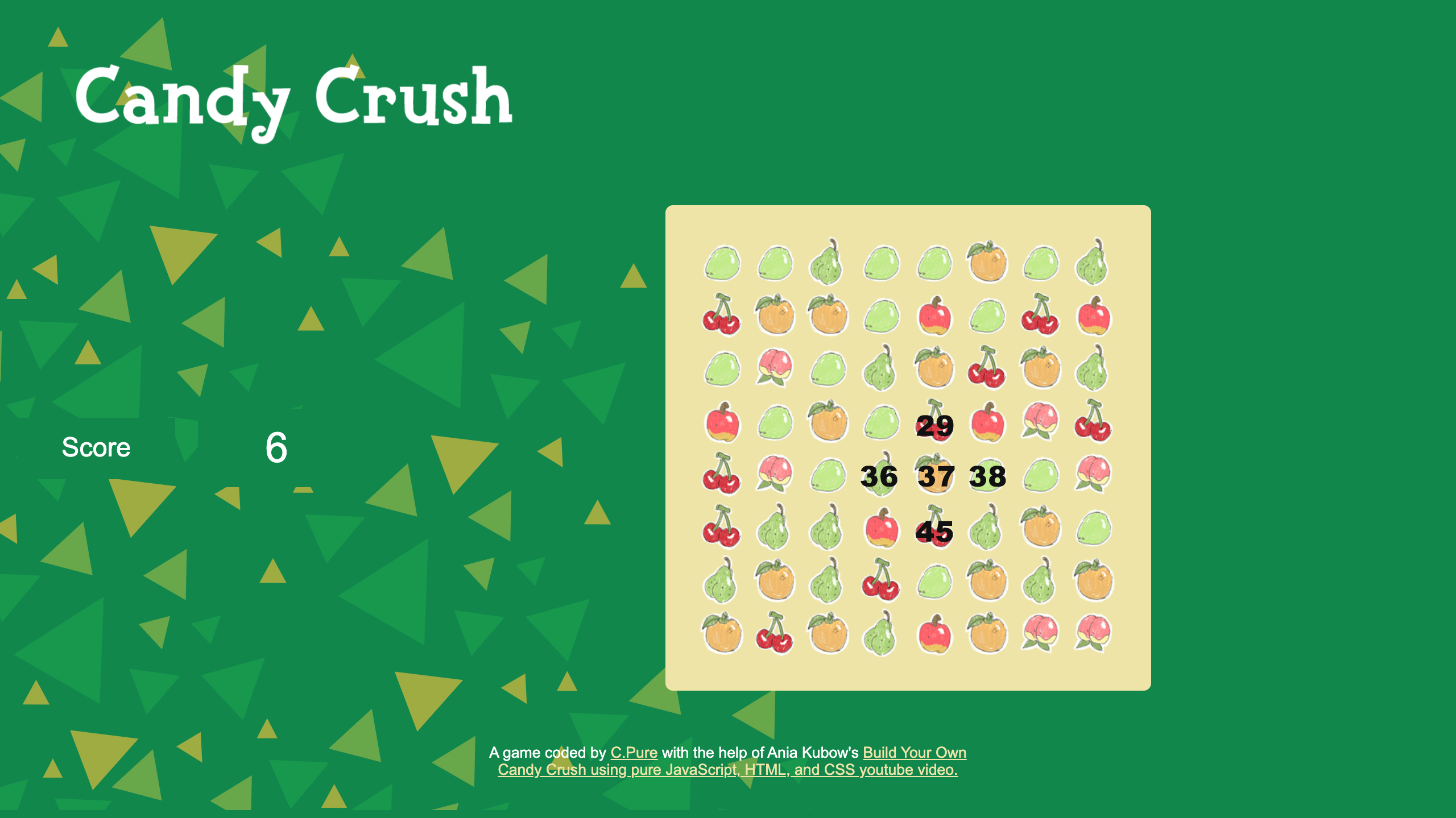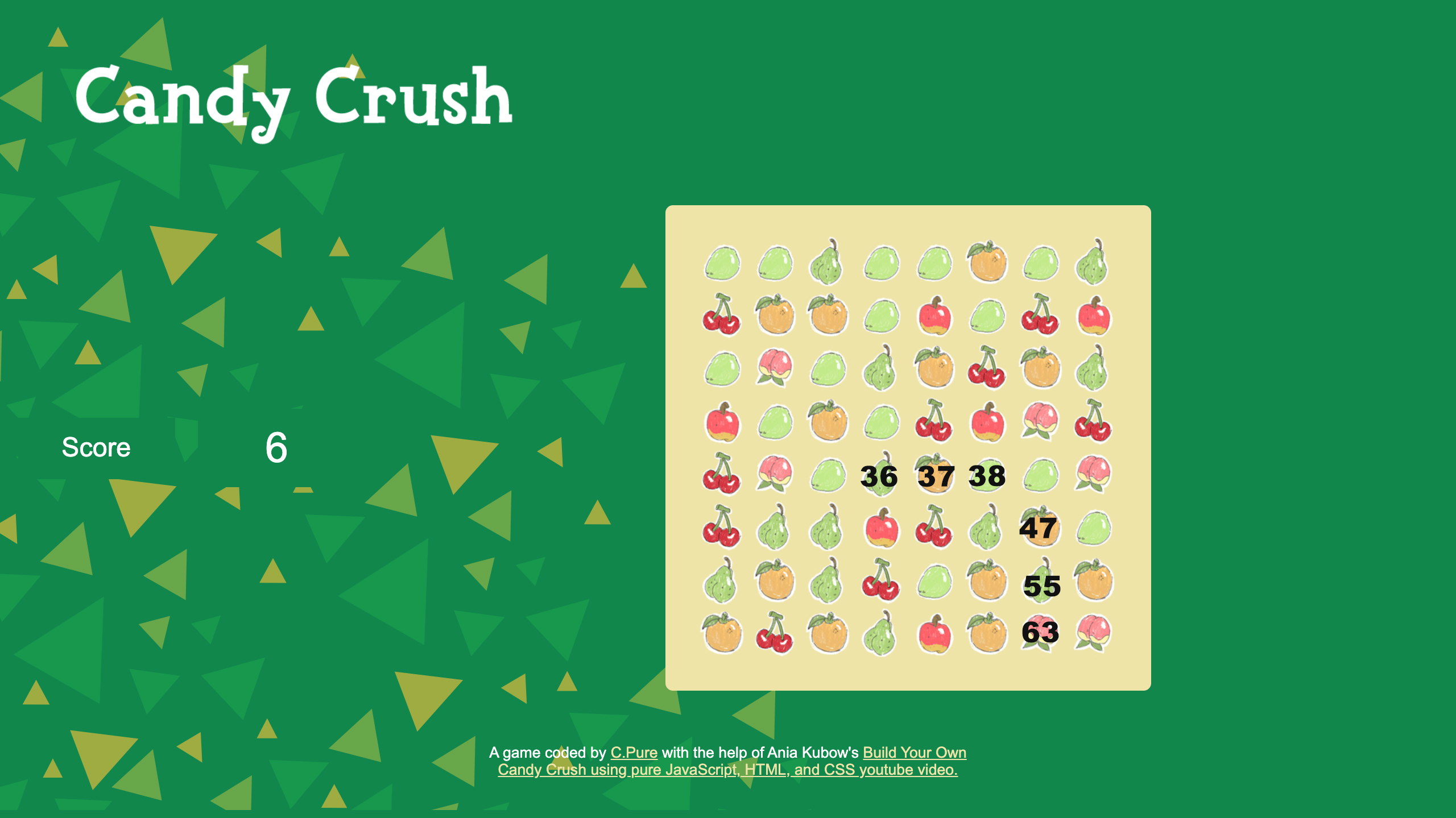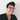# Breaking Down Ania Kabow’s Build Your Own Candy Crush using JS Part 3 - Validate Moves & Checking for Matches

August 14, 2020

This post first appeared on my Dev.to blog on August 14, 2020. This is the third post in the series. You can read Part 2 here.

Continuing on from part 2 of this technical breakdown, the next task in the game is to check for valid moves and matches.

## Valid Moves

First we create an array of all the valid moves.

``let validMoves = [ ]``

Inside the array we add the following

#### squareIdBeingDragged + 1

This as Ania explains will make the move to the left or right of the dragged square valid. For example if I drag a square with an id of 67 it can move one over and switch with a square with an id of 66 or 68.

#### squareIdBeingDragged + width

This item will make moving one up or down valid. This takes the ID and will subtracts or add the width which is 8. Below is a visual representation of this array and the valid moves we are creating.Final Array

``````let validMoves = [
squareIdBeingDragged - 1,
squareIdBeingDragged - width,
squareIdBeingDragged + 1,
squareIdBeingDragged + width

]``````

## Defining a valid move

We used the ‘includes() method’ to validate these moves in the array. The includes method determines whether a string contains the specified characters in another string. This will return a boolean value of true or false when assessed.

``let validMove = validMoves.includes(squareIdBeingReplaced)``

This statement reads if the ID value of the replaced square is in our validMoves array the statement is true, making it a valid move.

Next, we will create an if statement to check that the ID of the squareIdBeingReplaced exists and that the move is valid. If both statements return back “truthy” we clear the value of the square being replaced. To do that we use the null value which is an intentional absence of an object value.

If it is not a valid move we will return squareIdBeingReplaced and squareIdBeingDragged. We do this by setting both to its original color. And finally if a square just has no where to go, like it’s on the edge of the board, it will just return to its original space.

``````if (squareIdBeingReplaced && validMove) {
squareIdBeingReplaced = null
} else if (squareIdBeingReplaced && !validMove) {
squares [squareIdBeingReplaced].style.backgroundColor = colorBeingReplaced
squares [squareIdBeingDragged].style.backgroundColor = colorBeingDragged
} else squares [squareIdBeingDragged].style.backgroundColor = colorBeingDragged

}``````

The final code should be within the dragEnd function as so:

``````function dragEnd() {
console.log(this.id, ‘dragend’)

let validMoves = [
squareIdBeingDragged - 1,
squareIdBeingDragged - width,
squareIdBeingDragged + 1,
squareIdBeingDragged + width
]

let validMove = validMoves.includes(squareIdBeingReplaced)

if (squareIdBeingReplaced && validMove) {
squareIdBeingReplaced = null
} else if (squareIdBeingReplaced && !validMove) {
squares [squareIdBeingReplaced].style.backgroundColor = colorBeingReplaced
squares [squareIdBeingDragged].style.backgroundColor = colorBeingDragged
} else squares [squareIdBeingDragged].style.backgroundColor = colorBeingDragged

}``````

## Checking For a Match

In Candy Crush you can get matches of 3, 4, and 5. We create a function called checkRowForThree and will use that logic for matches of four and five.

``````function checkRowForThree() {
}``````

Using a for loop we will loop through the possible three-square match up to index 61. The last possible square we could loop over in order to not break the game is 61. This loop will check 61, 62, and 63 match three colors.

``````function checkRowForThree() {
for(i = 0; i < 61; i++){

}
}``````

Inside of the for loop code block we define a row of three in a variable which is written as so:

``let rowOfThree = [i, i+1, i+2]``Each time we loop through this we want to grab the color of the first square and define it in a variable we titled decidedColor.

``let decidedColor = squares[i].style.backgroundColor``

And we will also define a blank space. A blank space is defined when the square background color is an empty string. We use a constant to declare the isBlank variable since we don’t want to update this later. We want this to always stay constant. Here is a link again to the post by Wes Bos - let VS const

``const isBlank = squares[i].style.backgroundColor === ‘ ’``

Still inside of the for loop we will define the logic using an if statement. We attach the every method to our rowOfThree array to check that every index in our array equals the color of our first square and also that it is not a blank square. If so we execute the code.

``````if (rowOfThree.every(index => squares[index].style.backgroundColor === decidedColor && !isBlank){

}``````

Inside this code block, for each match we want to set the background color to an empty string. We also made this match a score of plus three points.

Note: We had to make sure we added the score variable as a global variable at the top of our code. You can add it just underneath the const squares empty array.

``````document.addEventListener('DOMContentLoaded'), () => {
const grid = document.querySelector('.grid')
const width = 8
const squares = []
let score = 0``````
``````if (rowOfThree.every(index => squares[index].style.backgroundColor === decidedColor && !isBlank){
score += 3
rowOfThree.forEach(index => {
squares[index].style.backgroundColor = ''
})
}``````

We test the checkRowForThree code by invoking it. We see that it works but we would like it to execute constantly while playing and to do that we add a set interval function to the window.

The setInterval function takes four parameters a function to execute, code, delay, and any additional arguments. In this case we want to invoke the checkRowForThree function every 100 milliseconds.

One last thing to add to our checkRowForThree function is where a move is not valid. Since we did not tell the program that matches can not wrap the board to the next line and be considered a valid match of three.

We will create another const named notValid and make an array of all the IDs we don’t want the row to start at. Then we will use an if statement to say if an ID is one of the numbers in the notValid array that we continue on and not count those as possible options for a valid start to a row of three.

``````function checkRowForThree() {
for(i = 0; i < 61; i++){
let rowOfThree = [i, i+1, i+2]
let decidedColor = squares[i].style.backgroundColor
const isBlank = squares[i].style.backgroundColor === ‘ ’

const notValid = [6, 7, 14, 15, 22, 23, 30, 31, 38, 39, 46, 47, 54, 55 ]

if(notValid.includes(i)) continue

if (rowOfThree.every(index => squares[index].style.backgroundColor === decidedColor && !isBlank){
score += 3
rowOfThree.forEach(index => {
squares[index].style.backgroundColor = ''
})
}
}
}

//This will only invoke the function once when we start the game.
checkRowForThree();

//Set Interval will repeatedly check for matches
window.setInterval(function(){
checkRowForThree()
}, 100)
)``````

Now that we have that set we will created another function but this time to check for a column of three. Since we are now looking for a column match, our loop will stop at 47 and indexes in our array will add the width and width*2.

Note that we changed all of the code to say checkColumn or columnOf so that it represents what this function is doing.

``````function checkColumnForThree() {
for(i = 0; i < 47; i++){
let columnOfThree = [i, i+width, i+width*2]
let decidedColor = squares[i].style.backgroundColor
const isBlank = squares[i].style.backgroundColor === ‘ ’
if (columnOfThree.every(index => squares[index].style.backgroundColor === decidedColor && !isBlank){
score += 3
columnOfThree.forEach(index => {
squares[index].style.backgroundColor = ''
})
}
}
}

//This will only invoke the function once when we start the game.
checkColumnForThree();

//Set Interval will repeatedly check for matches
window.setInterval(function(){
checkRowForThree()
checkColumnForThree()
}, 100)
)``````

Now that we have a check for row and column we can use these same functions for checking for valid matches of 4 and valid matches of 5.

In each function we will have to update each name to reflect what it is doing for example checkRowForFour. We also updated the following

• for loop to stop at 60
• added i+3 to the rowOfFour variable.
• Updated the score to score += 4
• Lastly the notValid const needs to be updated by checking the indexes one square earlier as shown below. Again this is only for the rows.
``const notValid = [5, 6, 7, 13, 14, 15, 21, 22, 23, 29, 30, 31, 37, 38, 39, 45, 46, 47, 53, 54, 55 ]``

In the setInterval function we will add the new function calls to the list as so. We added the checkRow/Column For Four

``````window.setInterval(function(){
checkRowForFour()
checkColumnForFour()
checkRowForThree()
checkColumnForThree()
}, 100)``````

The final code for this section is below. In the video Ania covered how to do three and four and suggested to write match of 5 on our own since it uses the same principles. The next and last part of this series will be breaking down how to “Move Candies Down & Generate New Candies.”

``````function dragEnd() {
console.log(this.id, ‘dragend’)

let validMoves = [
squareIdBeingDragged - 1,
squareIdBeingDragged - width,
squareIdBeingDragged + 1,
squareIdBeingDragged + width
]

let validMove = validMoves.includes(squareIdBeingReplaced)

if (squareIdBeingReplaced && validMove) {
squareIdBeingReplaced = null
} else if (squareIdBeingReplaced && !validMove) {
squares [squareIdBeingReplaced].style.backgroundColor = colorBeingReplaced
squares [squareIdBeingDragged].style.backgroundColor = colorBeingDragged
} else squares [squareIdBeingDragged].style.backgroundColor = colorBeingDragged

}

// Check row for a match of 3
function checkRowForThree() {
for(i = 0; i < 61; i++){
let rowOfThree = [i, i+1, i+2]
let decidedColor = squares[i].style.backgroundColor
const isBlank = squares[i].style.backgroundColor === ‘ ’

const notValid = [6, 7, 14, 15, 22, 23, 30, 31, 38, 39, 46, 47, 54, 55 ]

if(notValid.includes(i)) continue

if (rowOfThree.every(index => squares[index].style.backgroundColor === decidedColor && !isBlank){
score += 3
rowOfThree.forEach(index => {
squares[index].style.backgroundColor = ''
})
}
}
}

// Check column for a match of 3
function checkColumnForThree() {
for(i = 0; i < 47; i++){
let columnOfThree = [i, i+width, i+width*2]
let decidedColor = squares[i].style.backgroundColor
const isBlank = squares[i].style.backgroundColor === ‘ ’
if (columnOfThree.every(index => squares[index].style.backgroundColor === decidedColor && !isBlank){
score += 3
columnOfThree.forEach(index => {
squares[index].style.backgroundColor = ''
})
}
}
}

// Check row for a match of 4
function checkRowForFour() {
for(i = 0; i < 60; i++){
let rowOfFour = [i, i+1, i+2, i+3]
let decidedColor = squares[i].style.backgroundColor
const isBlank = squares[i].style.backgroundColor === ‘ ’

const notValid = [5, 6, 7, 13, 14, 15, 21, 22, 23, 29, 30, 31, 37, 38, 39, 45, 46, 47, 53, 54, 55]

if(notValid.includes(i)) continue

if (rowOfFour.every(index => squares[index].style.backgroundColor === decidedColor && !isBlank){
score += 4
rowOfFour.forEach(index => {
squares[index].style.backgroundColor = ''
})
}
}
}

// Check column for a match of 4
function checkColumnForFour() {
for(i = 0; i < 39; i++){
let columnOfFour = [i, i+width, i+width*2, i+width*3]
let decidedColor = squares[i].style.backgroundColor
const isBlank = squares[i].style.backgroundColor === ‘ ’
if (columnOfFour.every(index => squares[index].style.backgroundColor === decidedColor && !isBlank){
score += 4
columnOfFour.forEach(index => {
squares[index].style.backgroundColor = ''
})
}
}
}

window.setInterval(function(){
checkRowForFour()
checkColumnForFour()
checkRowForThree()
checkColumnForThree()
}, 100)``````

## MDN web docs

### Topics Includes:

Each function and method above is linked to their respective MDN web doc pages. This concludes the third part in this series of Breaking Down Ania Kabow’s Candy Crush video.

If there are any errors in my syntax or grammar please shoot me a comment or message to let me know! This is my first technical blog post so I want to make sure I’m sharing the best information possible.Written by Courtney Pure. Currently lives and works in Long Island, New York. She builds things with code and makes things move with animation and motion graphics! You should follow her on Twitter